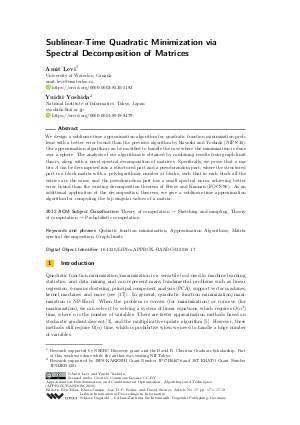Document# Sublinear-Time Quadratic Minimization via Spectral Decomposition of Matrices

### Authors Amit Levi, Yuichi Yoshida## File

LIPIcs.APPROX-RANDOM.2018.17.pdf
• Filesize: 0.51 MB
• 19 pages

## Cite As

Amit Levi and Yuichi Yoshida. Sublinear-Time Quadratic Minimization via Spectral Decomposition of Matrices. In Approximation, Randomization, and Combinatorial Optimization. Algorithms and Techniques (APPROX/RANDOM 2018). Leibniz International Proceedings in Informatics (LIPIcs), Volume 116, pp. 17:1-17:19, Schloss Dagstuhl - Leibniz-Zentrum für Informatik (2018)
https://doi.org/10.4230/LIPIcs.APPROX-RANDOM.2018.17

## Abstract

We design a sublinear-time approximation algorithm for quadratic function minimization problems with a better error bound than the previous algorithm by Hayashi and Yoshida (NIPS'16). Our approximation algorithm can be modified to handle the case where the minimization is done over a sphere. The analysis of our algorithms is obtained by combining results from graph limit theory, along with a novel spectral decomposition of matrices. Specifically, we prove that a matrix A can be decomposed into a structured part and a pseudorandom part, where the structured part is a block matrix with a polylogarithmic number of blocks, such that in each block all the entries are the same, and the pseudorandom part has a small spectral norm, achieving better error bound than the existing decomposition theorem of Frieze and Kannan (FOCS'96). As an additional application of the decomposition theorem, we give a sublinear-time approximation algorithm for computing the top singular values of a matrix.

## Subject Classification

##### ACM Subject Classification
• Theory of computation → Sketching and sampling
• Theory of computation → Probabilistic computation
##### Keywords
• Qudratic function minimization
• Approximation Algorithms
• Matrix spectral decomposition
• Graph limits

## Metrics

• Access Statistics
• Total Accesses (updated on a weekly basis)
0

## References

1. Farid Alizadeh. Interior point methods in semidefinite programming with applications to combinatorial optimization. SIAM journal on Optimization, 5(1):13-51, 1995.2. Aharon Ben-Tal and Marc Teboulle. Hidden convexity in some nonconvex quadratically constrained quadratic programming. Mathematical Programming, 72(1):51-63, 1996.3. Léon Bottou. Stochastic learning. In Advanced Lectures on Machine Learning, pages 146-168. Springer, 2004.4. Stanislav Busygin. A new trust region technique for the maximum weight clique problem. Discrete Applied Mathematics, 154(15):2080-2096, 2006.5. Kenneth L. Clarkson, Elad Hazan, and David P. Woodruff. Sublinear optimization for machine learning. Journal of the ACM, 59(5):23:1-23:49, 2012.6. A Frieze and R Kannan. The regularity lemma and approximation schemes for dense problems. In Proceedings of the 37th Annual IEEE Symposium on Foundations of Computer Science (FOCS), pages 12-20, 1996.7. Alan Frieze and Ravi Kannan. Quick approximation to matrices and applications. Combinatorica, 19(2):175-220, 1999.8. Alan Frieze, Ravi Kannan, and Santosh Vempala. Fast monte-carlo algorithms for finding low-rank approximations. Journal of the ACM (JACM), 51(6):1025-1041, 2004.9. Walter Gander, Gene H Golub, and Urs von Matt. A constrained eigenvalue problem. Linear Algebra and its applications, 114:815-839, 1989.10. Kohei Hayashi and Yuichi Yoshida. Minimizing quadratic functions in constant time. In Proceedings of the 30th Annual Conference on Neural Information Processing Systems (NIPS), pages 2217-2225, 2016.11. Kohei Hayashi and Yuichi Yoshida. Fitting low-rank tensors in constant time. In Proceedings of the 31th Annual Conference on Neural Information Processing Systems (NIPS), pages 2473-2481, 2017.12. Elad Hazan and Tomer Koren. A linear-time algorithm for trust region problems. Mathematical Programming, 158(1-2):363-381, 2016.13. L Lovász. Large Networks and Graph Limits. American Mathematical Society, 2012.14. László Lovász and Balázs Szegedy. Limits of dense graph sequences. Journal of Combinatorial Theory, Series B, 96(6):933-957, 2006.15. David G. Luenberger. Optimization by Vector Space Methods. John Wiley &Sons, Inc., New York, NY, USA, 1st edition, 1997.16. Claire Mathieu and Warren Schudy. Yet another algorithm for dense max cut: go greedy. In Proceedings of the 19th Annual ACM-SIAM Symposium on Discrete Algorithms (SODA), pages 176-182, 2008.17. Kevin P Murphy. Machine learning: a probabilistic perspective. The MIT Press, 2012.18. Yurii Nesterov. A method of solving a convex programming problem with convergence rate o (1/k2). In Soviet Mathematics Doklady, volume 27, pages 372-376, 1983.19. Yurii Nesterov and Arkadii Nemirovskii. Interior-point polynomial algorithms in convex programming. SIAM, 1994.20. Vladimir Nikiforov. Cut-norms and spectra of matrices. arXiv:0912.0336, 2009.21. Anthony L. Peressini, Francis E. Sullivan, and J. J. Jr. Uhl. The Mathematics of Nonlinear Programming. Springer, 1993.22. Joel A Tropp. An introduction to matrix concentration inequalities. Foundations and Trends registered in Machine Learning, 8:1-230, 2015.23. Yinyu Ye and Shuzhong Zhang. New results on quadratic minimization. SIAM Journal on Optimization, 14(1):245-267, 2003.24. Hongchao Zhang, Andrew R Conn, and Katya Scheinberg. A derivative-free algorithm for least-squares minimization. SIAM Journal on Optimization, 20(6):3555-3576, 2010.X

Feedback for Dagstuhl Publishing